# Rotations of order 6 with reflections, no hexagon or 6-pointed star

The remaining patterns are numbered 112, which implies that one more further question needs to the answered.

The first group are examples of patterns with an equilateral triangle. An example of two of these are: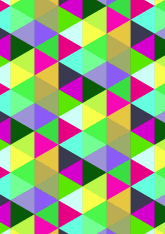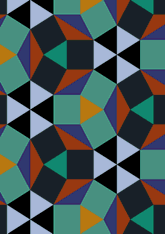The number in this group is 29, which is 25% of the total. Now make the final selection, see page.
The second group are examples of patterns which have a 3-pointed star. An example of two of these are: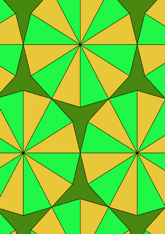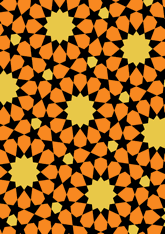The number in this group is 27, which is 24% of the total. Now make the final selection, see page.
The third group are examples of patterns with 12-pointed star. An example of two of these are: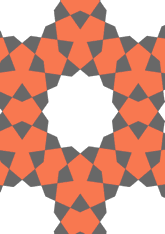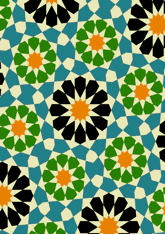The number in this group is 45, which is 40% of the total. Now make the final selection, see page.
The last group are examples of patterns with none of the above. An example of two of these are: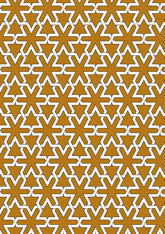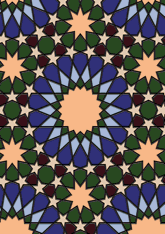The number in this group is 11, which is 9% of the total. Now make the final selection, see page.

v53

Tiling Search was created by Brian Wichmann, and is maintained by MIT Libraries, through support from the Aga Khan Documentation Center.

Also supported by Performant Software Solutions LLC.